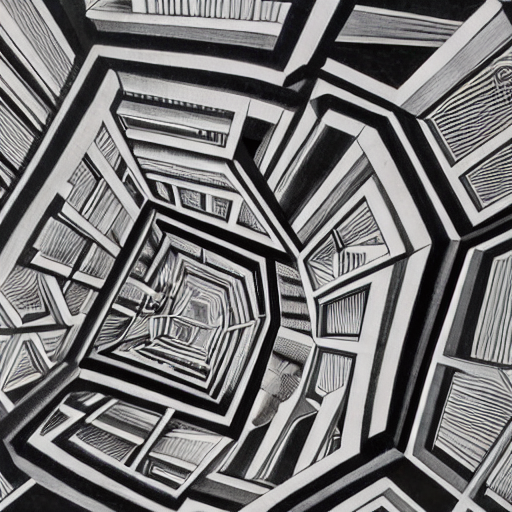### Derivative tricks

Nonstandard derivative exercises, used for a calculus class I'm teaching.

## Using this document

I recommend you think for 1-3 minutes after getting stuck before viewing a hint, and 10-15 minutes before viewing giving up and viewing the solution.

Pay attention to the lower and upper bounds! There's no honor in staring blankly at a problem for 30 minutes when you're stuck, and likewise viewing solutions prematurely is inefficient.

## Exercises

### Inverse and Implicit Differentiation

Using the fact that $(e^x)' = e^x$ and $e^{\ln x} = x$ find $(\ln x)'$

Hint

Differentiate both sides of $e^{\ln x} = x$ then solve for $(\ln x)'$

Solution

We know

$e^{\ln x} = x$

Taking the derivative of both sides and using the chain rule gives

$(\ln x)'e^{\ln x} = 1$

Since $e^{\ln x} = x$ dividing by $x$ isolates $(\ln x)'$ to give

$(\ln x)' = 1/x$

### Logarithmic Differentiation

Derive the product rule $(fg)' = f'g+g'f$ using the chain rule and logarithms.

Hint

Logarithms turn multiplication into addition, and we know how to handle addition (derivative of sum is sum of derivatives!)

Solution

Let $h = fg$ (or more verbosely $h(x) = f(x)g(x)$) then take the log of both sides

$\ln h = \ln f + \ln g$

Differentiate both sides, the chain rule and the fact that $(\ln x)' = 1/x$ imply $(\ln f(x))' = f'(x)/f(x)$ giving

$\frac{h'}{h} = \frac{f'}{f} + \frac{g'}{g}$

Since $h = fg$ this becomes

$\frac{(fg)'}{fg} = \frac{f'}{f} + \frac{g'}{g}$

Solving for $(fg)'$ by multiplying both sides by $fg$ finally gives the familiar product rule

$(fg)' = f'g + g'f$

Generalize the product rule to a product $f_1\dots f_n$ of more than two functions.

Hint

Use the logarithm approach from the previous exercise.

Solution

Let $h = f_1\dots f_n$, take logs to get

$\ln h = \sum_{i=1}^n \ln f_i$

Take derivatives

$\frac{h'}{h} = \sum_{i=1}^n \frac{f_i'}{f_i}$

Multiply both sides by $h$ and substitute back in for $h$

$(f_1\dots f_n)' = f_1'(f_2\dots f_n) + f_2'(f_1f_3\dots f_n) + \dots$

Compute the following derivative

$\frac{d}{dx} \frac{x^5}{(1 - 10x)\sqrt{x^2 + 2}}$
Hint

Write

$y = \frac{x^5}{(1 - 10x)\sqrt{x^2 + 2}}$

and take the logarithm of both sides

Solution

Take the log of both sides and simplify

\begin{aligned} \ln y &= \ln\left(\frac{x^5}{(1 - 10x)\sqrt{x^2 + 2}}\right) \\ &= 5 \ln(x) - \ln(1 - 10x) - \frac 12 \ln(x^2 + 2) \end{aligned}

Take derivatives to get

$\frac{y'}{y} = 5\frac{1}{x} - \frac{-10}{1 - 10x} - \frac 12 \frac{2x}{x^2 + 2}$

Multiply by $y$ and substitute back in

$y' = \left(\frac{x^5}{(1 - 10x)\sqrt{x^2 + 2}}\right)\left(\frac{5}{x} - \frac{-10}{1 - 10x} - \frac 12 \frac{2x}{x^2 + 2}\right)$

### Deriving Identities

In probability, we need to compute the following sum to find the expected value of a random variable with a geometric distribution

$E(X) = \sum_{k=0}^\infty kq^kp$

Using the identity $\sum_{k=0}^\infty q^k = \frac{1}{1-q}$ find the sum above

Hint

Differentiate both sides of the identity with respect to $q$

Solution
$\frac{1}{1-q} = \sum_{k=0}^\infty q^k \substack{\frac{d}{dx} \\ \implies} \frac{1}{(1-q)^2} = \sum_{k=0}^\infty kq^{k-1}$

Multiply both sides by $q$ to turn $q^{k-1}$ into $q^k$, multiply by $p$ to get the desired sum

$\frac{qp}{(1-q)^2} = \sum_{k=0}^\infty kq^kp$

(Footnote about probability: since $q=1-p$ the expectation simplifies to $1/p - 1$.)